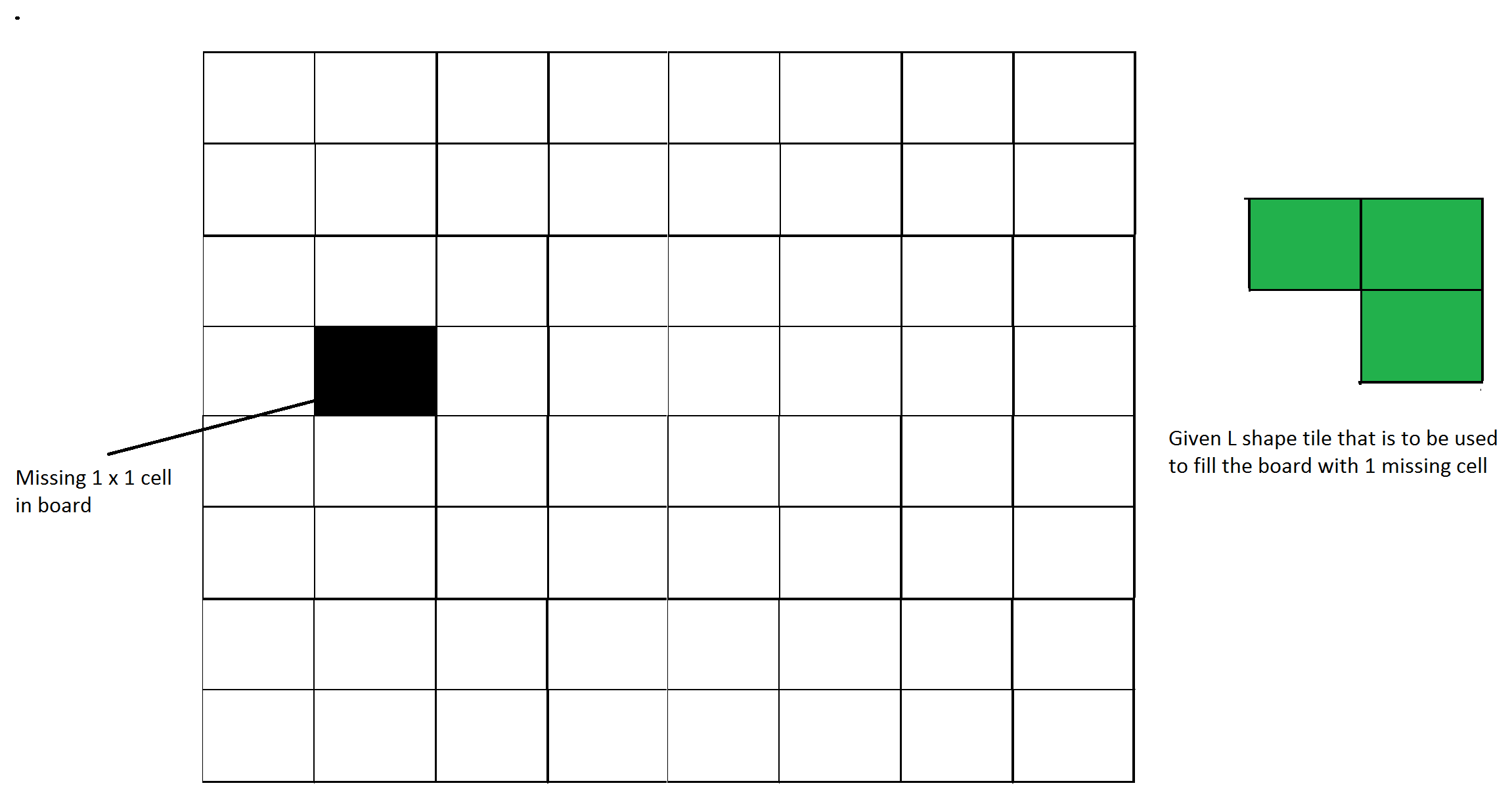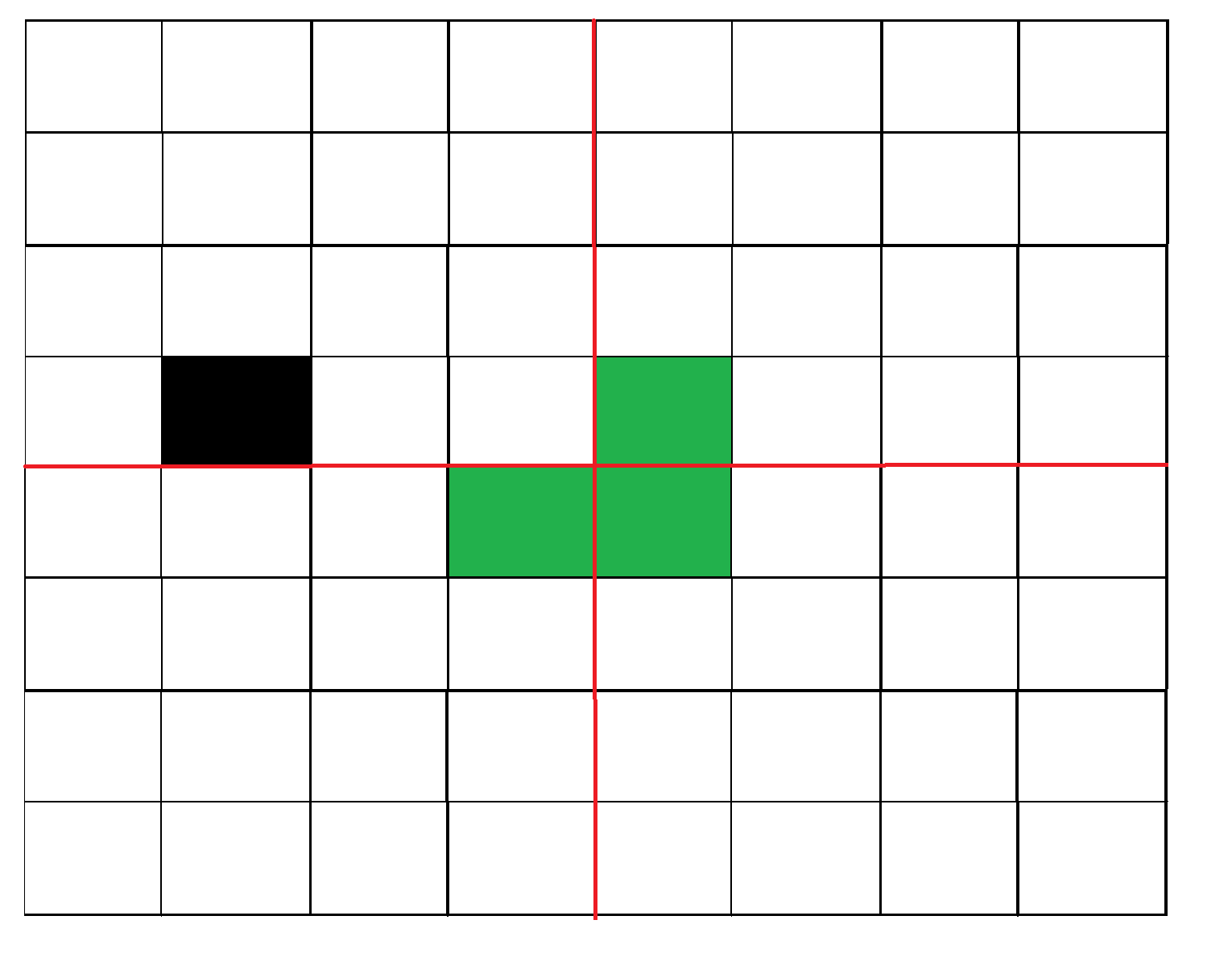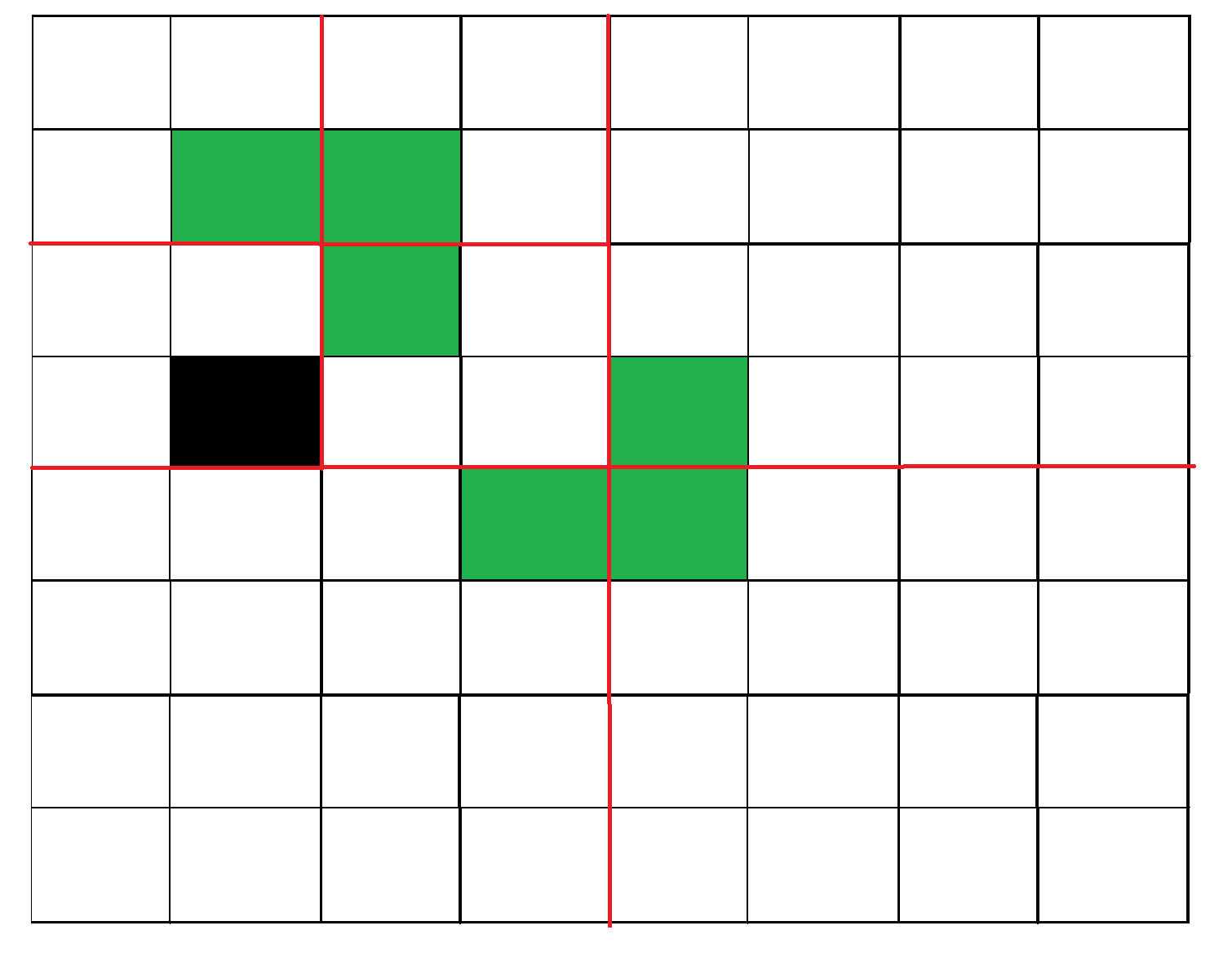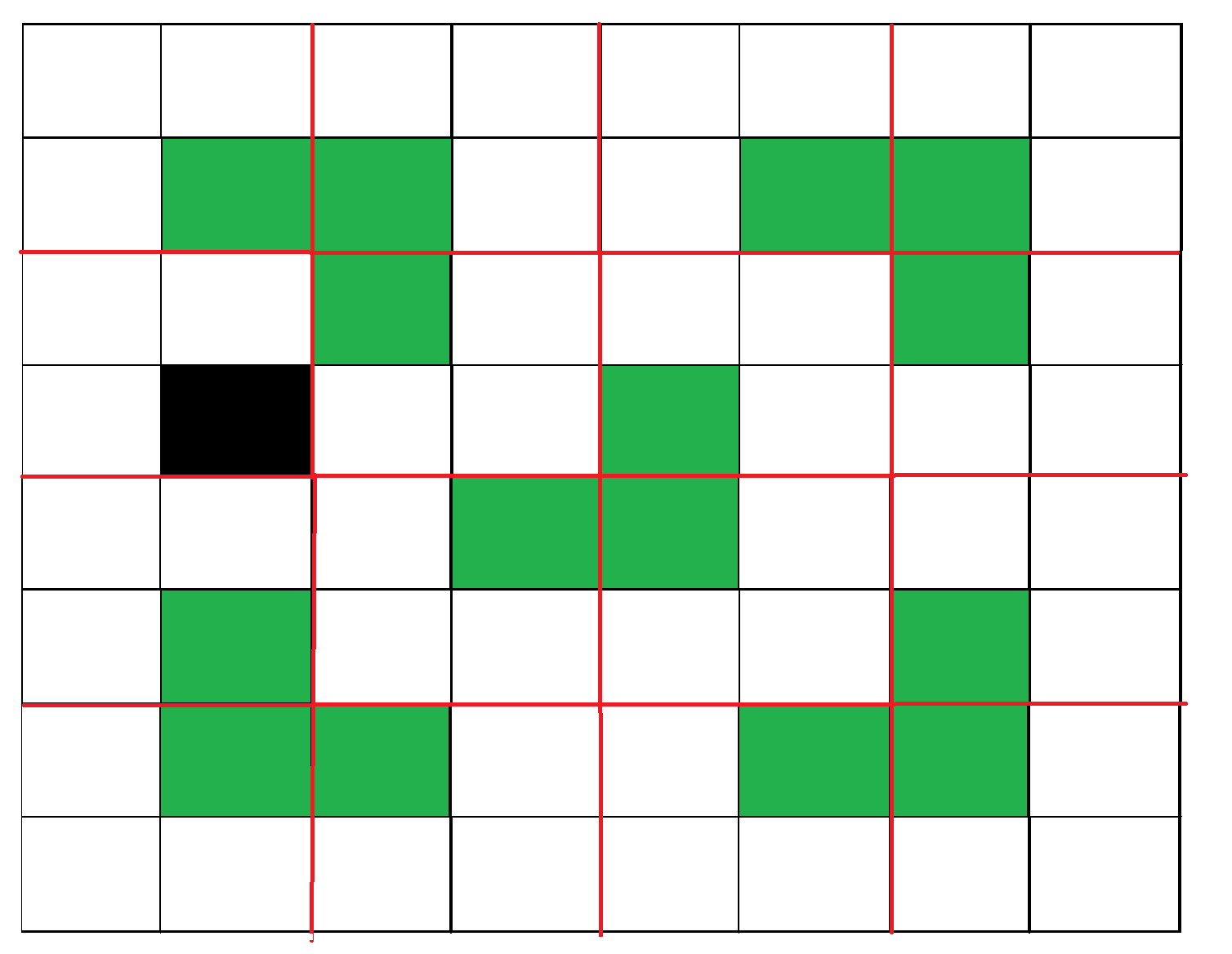GFG App
Open AppBrowser
Continue

# Tiling Problem using Divide and Conquer algorithm

Given a n by n board where n is of form 2k where k >= 1 (Basically n is a power of 2 with minimum value as 2). The board has one missing cell (of size 1 x 1). Fill the board using L shaped tiles. A L shaped tile is a 2 x 2 square with one cell of size 1×1 missing.Figure 1: An example input
This problem can be solved using Divide and Conquer. Below is the recursive algorithm.

```// n is size of given square, p is location of missing cell
Tile(int n, Point p)

1) Base case: n = 2, A 2 x 2 square with one cell missing is nothing
but a tile and can be filled with a single tile.

2) Place a L shaped tile at the center such that it does not cover
the n/2 * n/2 subsquare that has a missing square. Now all four
subsquares of size n/2 x n/2 have a missing cell (a cell that doesn't
need to be filled).  See figure 2 below.

3) Solve the problem recursively for following four. Let p1, p2, p3 and
p4 be positions of the 4 missing cells in 4 squares.
a) Tile(n/2, p1)
b) Tile(n/2, p2)
c) Tile(n/2, p3)
d) Tile(n/2, p3)```

The below diagrams show working of above algorithmFigure 2: After placing the first tileFigure 3: Recurring for the first subsquare.Figure 4: Shows the first step in all four subsquares.

Examples:

```Input :  size = 2 and mark coordinates = (0, 0)
Output :
-1      1
1       1
Coordinate (0, 0) is marked. So, no tile is there. In the remaining three positions,
a tile is placed with its number as 1.
Input : size = 4 and mark coordinates = (0, 0)
Output :
-1      3       2       2
3       3       1       2
4       1       1       5
4       4       5       5```

Below is the implementation of above idea:

## C++

 `// C++ program to place tiles` `#include ` `using` `namespace` `std;`   `int` `size_of_grid, b, a, cnt = 0;` `int` `arr;`   `// Placing tile at the given coordinates` `void` `place(``int` `x1, ``int` `y1, ``int` `x2, ` `           ``int` `y2, ``int` `x3, ``int` `y3)` `{` `    ``cnt++;` `    ``arr[x1][y1] = cnt;` `    ``arr[x2][y2] = cnt;` `    ``arr[x3][y3] = cnt;` `}` `// Quadrant names` `// 1   2` `// 3   4`   `// Function based on divide and conquer` `int` `tile(``int` `n, ``int` `x, ``int` `y)` `{` `    ``int` `r, c;` `    ``if` `(n == 2) {` `        ``cnt++;` `        ``for` `(``int` `i = 0; i < n; i++) {` `            ``for` `(``int` `j = 0; j < n; j++) {` `                ``if` `(arr[x + i][y + j] == 0) {` `                    ``arr[x + i][y + j] = cnt;` `                ``}` `            ``}` `        ``}` `        ``return` `0;` `    ``}` `    ``// finding hole location` `    ``for` `(``int` `i = x; i < x + n; i++) {` `        ``for` `(``int` `j = y; j < y + n; j++) {` `            ``if` `(arr[i][j] != 0)` `                ``r = i, c = j;` `        ``}` `    ``}`   `    ``// If missing tile is 1st quadrant` `    ``if` `(r < x + n / 2 && c < y + n / 2)` `        ``place(x + n / 2, y + (n / 2) - 1, x + n / 2,` `              ``y + n / 2, x + n / 2 - 1, y + n / 2);`   `    ``// If missing Tile is in 3rd quadrant` `    ``else` `if` `(r >= x + n / 2 && c < y + n / 2)` `        ``place(x + (n / 2) - 1, y + (n / 2), x + (n / 2),` `              ``y + n / 2, x + (n / 2) - 1, y + (n / 2) - 1);`   `    ``// If missing Tile is in 2nd quadrant` `    ``else` `if` `(r < x + n / 2 && c >= y + n / 2)` `        ``place(x + n / 2, y + (n / 2) - 1, x + n / 2,` `              ``y + n / 2, x + n / 2 - 1, y + n / 2 - 1);`   `    ``// If missing Tile is in 4th quadrant` `    ``else` `if` `(r >= x + n / 2 && c >= y + n / 2)` `        ``place(x + (n / 2) - 1, y + (n / 2), x + (n / 2),` `              ``y + (n / 2) - 1, x + (n / 2) - 1,` `              ``y + (n / 2) - 1);`   `    ``// dividing it again in 4 quadrants` `    ``tile(n / 2, x, y + n / 2);` `    ``tile(n / 2, x, y);` `    ``tile(n / 2, x + n / 2, y);` `    ``tile(n / 2, x + n / 2, y + n / 2);`   `    ``return` `0;` `}` `// Driver program to test above function` `int` `main()` `{` `    ``// size of box` `    ``size_of_grid = 8;` `    ``memset``(arr, 0, ``sizeof``(arr));` `    ``// Coordinates which will be marked` `    ``a = 0, b = 0;` `    ``// Here tile can not be placed` `    ``arr[a][b] = -1;` `    ``tile(size_of_grid, 0, 0);` `    ``// The grid is` `    ``for` `(``int` `i = 0; i < size_of_grid; i++) {` `        ``for` `(``int` `j = 0; j < size_of_grid; j++)` `            ``cout << arr[i][j] << ``" \t"``;` `        ``cout << ``"\n"``;` `    ``}` `}`

## Java

 `// Java program to place tiles` `public` `class` `GFG` `{` `  ``static` `int` `size_of_grid, b, a, cnt = ``0``;` `  ``static` `int``[][] arr = ``new` `int``[``128``][``128``];`   `  ``// Placing tile at the given coordinates` `  ``static` `void` `place(``int` `x1, ``int` `y1, ``int` `x2, ` `                    ``int` `y2, ``int` `x3, ``int` `y3)` `  ``{` `    ``cnt++;` `    ``arr[x1][y1] = cnt;` `    ``arr[x2][y2] = cnt;` `    ``arr[x3][y3] = cnt;` `  ``}` `  ``// Quadrant names` `  ``// 1   2` `  ``// 3   4`   `  ``// Function based on divide and conquer` `  ``static` `int` `tile(``int` `n, ``int` `x, ``int` `y)` `  ``{` `    ``int` `r = ``0``, c = ``0``;` `    ``if` `(n == ``2``) ` `    ``{` `      ``cnt++;` `      ``for` `(``int` `i = ``0``; i < n; i++) ` `      ``{` `        ``for` `(``int` `j = ``0``; j < n; j++)` `        ``{` `          ``if` `(arr[x + i][y + j] == ``0``)` `          ``{` `            ``arr[x + i][y + j] = cnt;` `          ``}` `        ``}` `      ``}` `      ``return` `0``;` `    ``}`   `    ``// finding hole location` `    ``for` `(``int` `i = x; i < x + n; i++)` `    ``{` `      ``for` `(``int` `j = y; j < y + n; j++) ` `      ``{` `        ``if` `(arr[i][j] != ``0``)` `        ``{` `          ``r = i;` `          ``c = j;` `        ``}`   `      ``}` `    ``}`   `    ``// If missing tile is 1st quadrant` `    ``if` `(r < x + n / ``2` `&& c < y + n / ``2``)` `      ``place(x + n / ``2``, y + (n / ``2``) - ``1``, x + n / ``2``,` `            ``y + n / ``2``, x + n / ``2` `- ``1``, y + n / ``2``);`   `    ``// If missing Tile is in 3rd quadrant` `    ``else` `if` `(r >= x + n / ``2` `&& c < y + n / ``2``)` `      ``place(x + (n / ``2``) - ``1``, y + (n / ``2``), x + (n / ``2``),` `            ``y + n / ``2``, x + (n / ``2``) - ``1``, y + (n / ``2``) - ``1``);`   `    ``// If missing Tile is in 2nd quadrant` `    ``else` `if` `(r < x + n / ``2` `&& c >= y + n / ``2``)` `      ``place(x + n / ``2``, y + (n / ``2``) - ``1``, x + n / ``2``,` `            ``y + n / ``2``, x + n / ``2` `- ``1``, y + n / ``2` `- ``1``);`   `    ``// If missing Tile is in 4th quadrant` `    ``else` `if` `(r >= x + n / ``2` `&& c >= y + n / ``2``)` `      ``place(x + (n / ``2``) - ``1``, y + (n / ``2``), x + (n / ``2``),` `            ``y + (n / ``2``) - ``1``, x + (n / ``2``) - ``1``,` `            ``y + (n / ``2``) - ``1``);`   `    ``// dividing it again in 4 quadrants` `    ``tile(n / ``2``, x, y + n / ``2``);` `    ``tile(n / ``2``, x, y);` `    ``tile(n / ``2``, x + n / ``2``, y);` `    ``tile(n / ``2``, x + n / ``2``, y + n / ``2``);  ` `    ``return` `0``;` `  ``} `   `  ``// Driver code` `  ``public` `static` `void` `main(String[] args)` `  ``{`   `    ``// size of box` `    ``size_of_grid = ``8``;`   `    ``// Coordinates which will be marked` `    ``a = ``0``; b = ``0``;`   `    ``// Here tile can not be placed` `    ``arr[a][b] = -``1``;` `    ``tile(size_of_grid, ``0``, ``0``);`   `    ``// The grid is` `    ``for` `(``int` `i = ``0``; i < size_of_grid; i++) ` `    ``{` `      ``for` `(``int` `j = ``0``; j < size_of_grid; j++)` `        ``System.out.print(arr[i][j] + ``" "``);` `      ``System.out.println();;` `    ``}` `  ``}` `}`   `// This code is contributed by divyeshrabadiya07.`

## C#

 `// C# program to place tiles` `using` `System;` `class` `GFG` `{` `    `  `    ``static` `int` `size_of_grid, b, a, cnt = 0;` `    ``static` `int``[,] arr = ``new` `int``[128, 128];` `     `  `    ``// Placing tile at the given coordinates` `    ``static` `void` `place(``int` `x1, ``int` `y1, ``int` `x2, ` `               ``int` `y2, ``int` `x3, ``int` `y3)` `    ``{` `        ``cnt++;` `        ``arr[x1, y1] = cnt;` `        ``arr[x2, y2] = cnt;` `        ``arr[x3, y3] = cnt;` `    ``}` `    ``// Quadrant names` `    ``// 1   2` `    ``// 3   4` `     `  `    ``// Function based on divide and conquer` `    ``static` `int` `tile(``int` `n, ``int` `x, ``int` `y)` `    ``{` `        ``int` `r = 0, c = 0;` `        ``if` `(n == 2) ` `        ``{` `            ``cnt++;` `            ``for` `(``int` `i = 0; i < n; i++) ` `            ``{` `                ``for` `(``int` `j = 0; j < n; j++)` `                ``{` `                    ``if` `(arr[x + i, y + j] == 0)` `                    ``{` `                        ``arr[x + i, y + j] = cnt;` `                    ``}` `                ``}` `            ``}` `            ``return` `0;` `        ``}` `      `  `        ``// finding hole location` `        ``for` `(``int` `i = x; i < x + n; i++)` `        ``{` `            ``for` `(``int` `j = y; j < y + n; j++) ` `            ``{` `                ``if` `(arr[i, j] != 0)` `                ``{` `                    ``r = i;` `                    ``c = j;` `                ``}` `                    `  `            ``}` `        ``}` `     `  `        ``// If missing tile is 1st quadrant` `        ``if` `(r < x + n / 2 && c < y + n / 2)` `            ``place(x + n / 2, y + (n / 2) - 1, x + n / 2,` `                  ``y + n / 2, x + n / 2 - 1, y + n / 2);` `     `  `        ``// If missing Tile is in 3rd quadrant` `        ``else` `if` `(r >= x + n / 2 && c < y + n / 2)` `            ``place(x + (n / 2) - 1, y + (n / 2), x + (n / 2),` `                  ``y + n / 2, x + (n / 2) - 1, y + (n / 2) - 1);` `     `  `        ``// If missing Tile is in 2nd quadrant` `        ``else` `if` `(r < x + n / 2 && c >= y + n / 2)` `            ``place(x + n / 2, y + (n / 2) - 1, x + n / 2,` `                  ``y + n / 2, x + n / 2 - 1, y + n / 2 - 1);` `     `  `        ``// If missing Tile is in 4th quadrant` `        ``else` `if` `(r >= x + n / 2 && c >= y + n / 2)` `            ``place(x + (n / 2) - 1, y + (n / 2), x + (n / 2),` `                  ``y + (n / 2) - 1, x + (n / 2) - 1,` `                  ``y + (n / 2) - 1);` `     `  `        ``// dividing it again in 4 quadrants` `        ``tile(n / 2, x, y + n / 2);` `        ``tile(n / 2, x, y);` `        ``tile(n / 2, x + n / 2, y);` `        ``tile(n / 2, x + n / 2, y + n / 2);  ` `        ``return` `0;` `    ``} `   `  ``// Driver code` `  ``static` `void` `Main() ` `  ``{` `    `  `    ``// size of box` `    ``size_of_grid = 8;` `    `  `    ``// Coordinates which will be marked` `    ``a = 0; b = 0;` `    `  `    ``// Here tile can not be placed` `    ``arr[a, b] = -1;` `    ``tile(size_of_grid, 0, 0);` `    `  `    ``// The grid is` `    ``for` `(``int` `i = 0; i < size_of_grid; i++) ` `    ``{` `        ``for` `(``int` `j = 0; j < size_of_grid; j++)` `            ``Console.Write(arr[i,j] + ``" "``);` `        ``Console.WriteLine();` `    ``}` `  ``}` `}`   `// This code is contributed by divyesh072019.`

## Python3

 `# Python3 program to place tiles` `size_of_grid ``=` `0` `b ``=` `0` `a ``=` `0` `cnt ``=` `0` `arr ``=` `[[``0` `for` `i ``in` `range``(``128``)] ``for` `j ``in` `range``(``128``)]`   `def` `place(x1, y1, x2, y2, x3, y3):` `    ``global` `cnt` `    ``cnt ``+``=` `1` `    ``arr[x1][y1] ``=` `cnt;` `    ``arr[x2][y2] ``=` `cnt;` `    ``arr[x3][y3] ``=` `cnt;` `    `  `def` `tile(n, x, y):` `    ``global` `cnt` `    ``r ``=` `0` `    ``c ``=` `0` `    ``if` `(n ``=``=` `2``):` `        ``cnt ``+``=` `1` `        ``for` `i ``in` `range``(n):` `            ``for` `j ``in` `range``(n):` `                ``if``(arr[x ``+` `i][y ``+` `j] ``=``=` `0``):` `                    ``arr[x ``+` `i][y ``+` `j] ``=` `cnt` `        ``return` `0``;    ` `    ``for` `i ``in` `range``(x, x ``+` `n):` `        ``for` `j ``in` `range``(y, y ``+` `n):` `            ``if` `(arr[i][j] !``=` `0``):` `                ``r ``=` `i` `                ``c ``=` `j  ` `    ``if` `(r < x ``+` `n ``/` `2` `and` `c < y ``+` `n ``/` `2``):` `        ``place(x ``+` `int``(n ``/` `2``), y ``+` `int``(n ``/` `2``) ``-` `1``, x ``+` `int``(n ``/` `2``), y ``+` `int``(n ``/` `2``), x ``+` `int``(n ``/` `2``) ``-` `1``, y ``+` `int``(n ``/` `2``))` `    `  `    ``elif``(r >``=` `x ``+` `int``(n ``/` `2``) ``and` `c < y ``+` `int``(n ``/` `2``)):` `        ``place(x ``+` `int``(n ``/` `2``) ``-` `1``, y ``+` `int``(n ``/` `2``), x ``+` `int``(n ``/` `2``), y ``+` `int``(n ``/` `2``), x ``+` `int``(n ``/` `2``) ``-` `1``, y ``+` `int``(n ``/` `2``) ``-` `1``)` `    `  `    ``elif``(r < x ``+` `int``(n ``/` `2``) ``and` `c >``=` `y ``+` `int``(n ``/` `2``)):` `        ``place(x ``+` `int``(n ``/` `2``), y ``+` `int``(n ``/` `2``) ``-` `1``, x ``+` `int``(n ``/` `2``), y ``+` `int``(n ``/` `2``), x ``+` `int``(n ``/` `2``) ``-` `1``, y ``+` `int``(n ``/` `2``) ``-` `1``)` `    `  `    ``elif``(r >``=` `x ``+` `int``(n ``/` `2``) ``and` `c >``=` `y ``+` `int``(n ``/` `2``)):` `        ``place(x ``+` `int``(n ``/` `2``) ``-` `1``, y ``+` `int``(n ``/` `2``), x ``+` `int``(n ``/` `2``), y ``+` `int``(n ``/` `2``) ``-` `1``, x ``+` `int``(n ``/` `2``) ``-` `1``, y ``+` `int``(n ``/` `2``) ``-` `1``)` `    `  `    ``tile(``int``(n ``/` `2``), x, y ``+` `int``(n ``/` `2``));` `    ``tile(``int``(n ``/` `2``), x, y);` `    ``tile(``int``(n ``/` `2``), x ``+` `int``(n ``/` `2``), y);` `    ``tile(``int``(n ``/` `2``), x ``+` `int``(n ``/` `2``), y ``+` `int``(n ``/` `2``)); ` `    `  `    ``return` `0`   `size_of_grid ``=` `8` `a ``=` `0` `b ``=` `0` `arr[a][b] ``=` `-``1` `tile(size_of_grid, ``0``, ``0``)`   `for` `i ``in` `range``(size_of_grid):` `    ``for` `j ``in` `range``(size_of_grid):` `        ``print``(arr[i][j], end``=``" "``)` `    ``print``()`   `# This code is contributed by rag2127`

## Javascript

 ``

Output

```-1     9     8     8     4     4     3     3
9     9     7     8     4     2     2     3
10     7     7     11     5     5     2     6
10     10     11     11     1     5     6     6
14     14     13     1     1     19     18     18
14     12     13     13     19     19     17     18
15     12     12     16     20     17     17     21
15     15     16     16     20     20     21     21     ```

Time Complexity:
Recurrence relation for above recursive algorithm can be written as below. C is a constant.
T(n) = 4T(n/2) + C
The above recursion can be solved using Master Method and time complexity is O(n2)

Space Complexity: O(n)

How does this work?
The working of Divide and Conquer algorithm can be proved using Mathematical Induction. Let the input square be of size 2k x 2k where k >=1.
Base Case: We know that the problem can be solved for k = 1. We have a 2 x 2 square with one cell missing.
Induction Hypothesis: Let the problem can be solved for k-1.
Now we need to prove  that the problem can be solved for k if it can be solved for k-1. For k, we put a L shaped tile in middle and we have four subsquares with dimension 2k-1 x 2k-1 as shown in figure 2 above. So if we can solve 4 subsquares, we can solve the complete square.

References:
http://www.comp.nus.edu.sg/~sanjay/cs3230/dandc.pdf